### Communication Systems Class 12th Physics Part Ii CBSE Solution

##### Question 1.Which of the following frequencies will be suitable for beyond-the horizon communication using sky waves?A. 10 kHzB. 10 MHzC. 1 GHzD. 1000 GHzAnswer:10 MHz frequency will be suitable for beyond the horizon communication using skywaves.10 KHz cannot be transmitted because the size of the antenna will be large for this apparently lower frequency.1 and 1000 GHz are very high frequency wave which will penetrate and so cannot be transmitted.Question 2.Frequencies in the UHF range normally propagate by means of:A. Ground waves.B. Sky waves.C. Surface waves.D. Space waves.Answer:The signals having Ultra High Frequency(UHF) can propagate through Space waves. These can neither travel along the trajectory of ground as shown in green nor can propagate by reflection in the ionosphere as shown in figure in red. The UHF signal propagates as shown in Blue in the figure.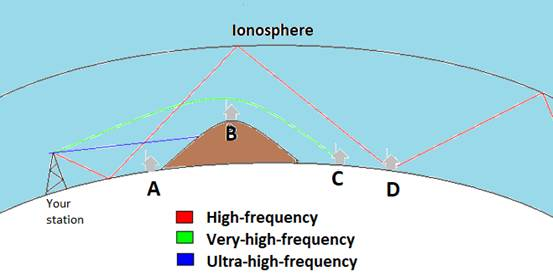Question 3.Digital signals(i) do not provide a continuous set of values,(ii) represent values as discrete steps,(iii) can utilize binary system, and(iv) can utilize decimal as well as binary systems.Which of the above statements are true?A. (i) and (ii) onlyB. (ii) and (iii) onlyC. (i), (ii) and (iii) but not (iv)D. All of (i), (ii), (iii) and (iv).Answer:Digital signal obviously use the binary signals i.e 0 and 1 only and no other signal other than these. It is also a signal that represents discontinuous values and it cannot utilize decimal values (0 to 9) which are continuous.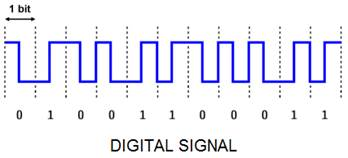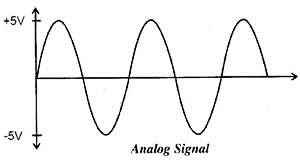Question 4.Is it necessary for a transmitting antenna to be at the same height as that of the receiving antenna for line-of-sight communication? A TV transmitting antenna is 81m tall. How much service area can it cover if the receiving antenna is at the ground level?Answer:No, it is not necessary for a transmitting antenna and the receiving antenna to be at the same height. Because in this specific case of transmission there is no physical obstruction between the transmitter and the receiver.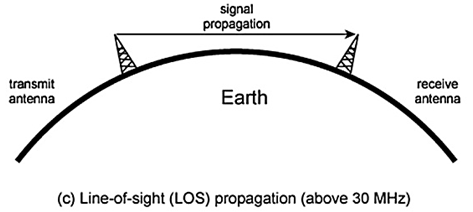(b) Height of the given antenna, h = 81 mRadius of earth, R = 6.4 × 106 mFor range, d = (2Rh)1/2, the service area of the antenna is given by the relation:A = π d2= π (2Rh)= 3.14 × 2 × 6.4 × 106 × 81 m2= 3255.55 × 106 m2~ 3256 km2[As 1km = 103 m , so 1km2 = 106 m2]Question 5.A carrier wave of peak voltage 12V is used to transmit a message signal. What should be the peak voltage of the modulating signal in order to have a modulation index of 75%?Answer:Amplitude of the carrier wave, Ac = 12 VModulation index, m = 75% = 0.75 (unitless as a ratio)Amplitude of the modulating wave = AmWe know for modulation index:m = Am/Ac⇒ Am = mAc⇒ Am = (0.75 × 12) V = 9VQuestion 6.A modulating signal is a square wave, as shown in Fig. 15.14.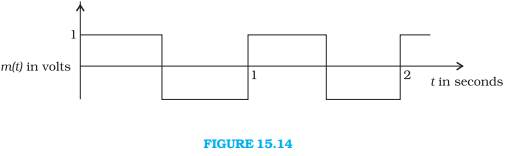The carrier wave is given by c (t) = 2sin(8πt) volts.(i) Sketch the amplitude modulated waveform(ii) What is the modulation index?Answer:It can be observed from the given modulating signal that the amplitude of the modulating signal, Am = 1 VIt is given that the carrier wave c (t) = 2sin(8πt) volts∴ Amplitude of the carrier wave, Ac = 2 VTime period of the modulating signal Tm = 1 sThe angular frequency of the modulating signal is calculated as:(t)m = 2π/Tm= 2π rad s-1 (as Tm = 1)……………….(i)The angular frequency of the carrier signal is calculated as:(t)m = 8π rad s-1 ……….(ii)From equations (i) and (ii), we get:(t)m = 4(t)cThe amplitude modulated waveform of the modulating signal is shown in the following figure.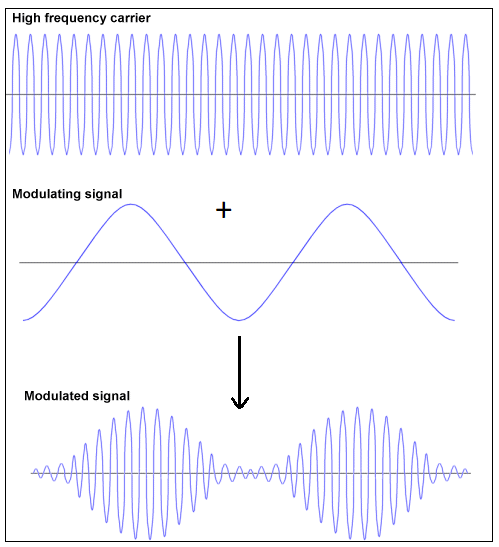(ii) Modulation index,m = Am/Ac = 1/2 = 0.5Question 7.For an amplitude modulated wave, the maximum amplitude is found to be 10V while the minimum amplitude is found to be 2V. Determine the modulation index, μ. What would be the value of μ if the minimum amplitude is zero volt?Answer:Maximum amplitude, Amax = 10 VMinimum amplitude, Amin = 2 VModulation index μ is given by the relation: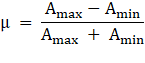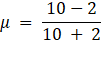⇒ μ = 8/12∴ μ = 0.67(b)If Amin= 0Then μ = Amax/Amax = 1Question 8.Due to economic reasons, only the upper sideband of an AM wave is transmitted, but at the receiving station, there is a facility for generating the carrier. Show that if a device is available which can multiply two signals, then it is possible to recover the modulating signal at the receiver station.Answer:Let ωc and ωs be the respective frequencies of the carrier and signal waves.Signal received at the receiving station, V = V1cos(ωc + ωs)tInstantaneous Voltage of the carrier wave, Vin = Vc cos ωctV.Vin = V1.Vc{cos(ωc + ωs)t. cos ωct}= ( V1.Vc)/2 {2cos(ωc + ωs)t. cosωct}= ( V1.Vc)/2 [cos{(ωc + ωs)t + ωct} + cos {{(ωc + ωs)t - ωct }= (V1.Vc)/2 [cos{(2ωc + ωs)t} + cos ωst ]As the receiving centre allows only high frequency signals to pass through it. Obstructs the low frequency signal ωs Thus, at the receiving station, one can record the modulating signal (V1Vc/2).cosωst , which is the signal frequency.

PDF FILE TO YOUR EMAIL IMMEDIATELY PURCHASE NOTES & PAPER SOLUTION. @ Rs. 50/- each (GST extra)

HINDI ENTIRE PAPER SOLUTION

MARATHI PAPER SOLUTION

SSC MATHS I PAPER SOLUTION

SSC MATHS II PAPER SOLUTION

SSC SCIENCE I PAPER SOLUTION

SSC SCIENCE II PAPER SOLUTION

SSC ENGLISH PAPER SOLUTION

SSC & HSC ENGLISH WRITING SKILL

HSC ACCOUNTS NOTES

HSC OCM NOTES

HSC ECONOMICS NOTES

HSC SECRETARIAL PRACTICE NOTES

# 2019 Board Paper Solution

HSC ENGLISH SET A 2019 21st February, 2019

HSC ENGLISH SET B 2019 21st February, 2019

HSC ENGLISH SET C 2019 21st February, 2019

HSC ENGLISH SET D 2019 21st February, 2019

SECRETARIAL PRACTICE (S.P) 2019 25th February, 2019

HSC XII PHYSICS 2019 25th February, 2019

CHEMISTRY XII HSC SOLUTION 27th, February, 2019

OCM PAPER SOLUTION 2019 27th, February, 2019

HSC MATHS PAPER SOLUTION COMMERCE, 2nd March, 2019

HSC MATHS PAPER SOLUTION SCIENCE 2nd, March, 2019

SSC ENGLISH STD 10 5TH MARCH, 2019.

HSC XII ACCOUNTS 2019 6th March, 2019

HSC XII BIOLOGY 2019 6TH March, 2019

HSC XII ECONOMICS 9Th March 2019

SSC Maths I March 2019 Solution 10th Standard11th, March, 2019

SSC MATHS II MARCH 2019 SOLUTION 10TH STD.13th March, 2019

SSC SCIENCE I MARCH 2019 SOLUTION 10TH STD. 15th March, 2019.

SSC SCIENCE II MARCH 2019 SOLUTION 10TH STD. 18th March, 2019.

SSC SOCIAL SCIENCE I MARCH 2019 SOLUTION20th March, 2019

SSC SOCIAL SCIENCE II MARCH 2019 SOLUTION, 22nd March, 2019

XII CBSE - BOARD - MARCH - 2019 ENGLISH - QP + SOLUTIONS, 2nd March, 2019

# HSCMaharashtraBoardPapers2020

(Std 12th English Medium)

HSC ECONOMICS MARCH 2020

HSC OCM MARCH 2020

HSC ACCOUNTS MARCH 2020

HSC S.P. MARCH 2020

HSC ENGLISH MARCH 2020

HSC HINDI MARCH 2020

HSC MARATHI MARCH 2020

HSC MATHS MARCH 2020

# SSCMaharashtraBoardPapers2020

(Std 10th English Medium)

English MARCH 2020

HindI MARCH 2020

Hindi (Composite) MARCH 2020

Marathi MARCH 2020

Mathematics (Paper 1) MARCH 2020

Mathematics (Paper 2) MARCH 2020

Sanskrit MARCH 2020

Sanskrit (Composite) MARCH 2020

Science (Paper 1) MARCH 2020

Science (Paper 2)

Geography Model Set 1 2020-2021

MUST REMEMBER THINGS on the day of Exam

Are you prepared? for English Grammar in Board Exam.

Paper Presentation In Board Exam

How to Score Good Marks in SSC Board Exams

Tips To Score More Than 90% Marks In 12th Board Exam

How to write English exams?

How to prepare for board exam when less time is left

How to memorise what you learn for board exam

No. 1 Simple Hack, you can try out, in preparing for Board Exam

How to Study for CBSE Class 10 Board Exams Subject Wise Tips?

JEE Main 2020 Registration Process – Exam Pattern & Important Dates

NEET UG 2020 Registration Process Exam Pattern & Important Dates

How can One Prepare for two Competitive Exams at the same time?

8 Proven Tips to Handle Anxiety before Exams!